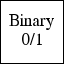Binary (0/1) ensemble ``` OP = OPBINARY(M,N,MODE) creates an M by N binary ensemble operator. When choosing MODE = 0 an explicit binary matrix is formed and used when applying the operator. Choosing MODE = 1 causes the operator to generate the above matrix on the fly, each time the operator is used. This mode can be used when dealing with very large matrices. ```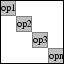Operator-diagonal operator ``` OPBLOCKDIAG(WEIGHTS, OP1, OP2, ...) creates a compound operator with the given weighted operators on the diagonal; DIAG([WEIGHT1*OP1, WEIGHT2*OP2, ...]). The WEIGHT parameter is optional and can either be a scalar or a vector. In case of a scalar, all operators are weighted equally. If only a single operator is given it is replicated as many times as there are weights. See also opFoG, opKron, opWindowedOp, opDictionary. ```Two-dimensional blurring operator ``` OPBLUR(M,N) creates an blurring operator for M by N images. This function is used for the GPSR-based test problems and is based on the implementation by Figueiredo, Nowak and Wright, 2007. This operator may soon be replaced by opConvolve2d. ```Restriction operator on matrix columns ``` OPCOLUMNRESTRCIT(M,N,IDX,TYPE), with TYPE = 'DISCARD', creates an operator that extracts the columns indicated by IDX from a given M by N matrix. The adjoint operator takes an M by length(IDX) matrix and outputs an M by N matrix with the columns filled by the given input matrix. Note that all input and output matrices are in vectorized from. When TYPE = 'ZERO', all columns that are not in IDX are zero-padded instead of discarded. See also opMask, opRestriction. ```One-dimensional convolution operator ``` OPCONVOLVE1D(KERNEL,OFFSET,CIRCULAR) creates a one-dimensional convolution operator with the KERNEL function. The length of the KERNEL vector must coincide with the signal length. OFFSET gives the index in the KERNEL vector to be taken as its center and is set to 1 by default (i.e. no shifting). The CIRCULAR flag indicates whether the signal is periodic. If set to any non-zero value convolution is wrapped around the vector endpoints. By default CIRCULAR is set to 1. ```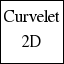Two-dimensional curvelet operator ``` OPCURVELET2D(M,N,NBSCALES,NBANGLES,TTYPE) creates a two-dimensional curvelet operator for M by N matrices. The curvelet transform is computed using the Curvelab code. The remaining three parameters are optional; NBSCALES gives the number of scales and is set to ceil(log2(min(M,N)) - 3) by default, as suggested by Curvelab. NBANGLES gives the number of angles at the second coarsest level which must be a multiple of four with a minimum of 8. By default NBANGLES is set to 16. TTYPE determines the type of transformation and is set to 'WRAP' by default. See also CURVELAB. ```One-dimensional discrete cosine transform (DCT) ``` OPDCT(N) creates a one-dimensional discrete cosine transform operator for vectors of length N. ```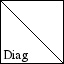Scaling operator (i.e., diagonal matrix). ``` OPDIAG(N,D) creates an operator for N by N diagonal matrix multiplication. The diagonal is given by D which can be either a vector of length N or a scalar. OPDIAG(D) is a shortcut for OPDIAG(LENGTH(D),D) and can be used instead. ```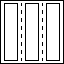Dictionary of concatenated operators ``` OPDICTIONARY(WEIGHTS, OP1, OP2, ...) creates a dictionary operator consisting of the concatenation of all operators; [WEIGHT1*OP1 | WEIGHT2*OP2 | ...]. The WEIGHT parameter is optional and can either be a scalar or a vector. In case of a scalar, all operators are weighted equally. See also opFoG, opBLockDiag. ```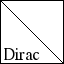Identity operator ``` OPDIRAC(N) creates the identity operator for vectors of length N. ```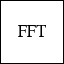One-dimensional fast Fourier transform (FFT). ``` OPFFT(N) create a one-dimensional normalized Fourier transform operator for vectors of length N. ```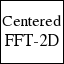Centralized two-dimensional fast Fourier transform (FFT). ``` OPFFT2C(M,N) creates a two-dimensional normalized Fourier Transform that operates on matrices of size M by N. The components are shifted to have to zero-frequency component in the center of the spectrum. ```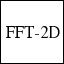Two-dimensional fast Fourier transform (FFT) ``` OPFFT2D(M,N) creats a two-dimensional normalized Fourier transform operator for matrices of size M by N. Input and output of the matrices is done in vectorized form. ```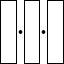Concatenate a sequence of operators into a single operator. ``` OPFOG(OP1,OP2,...OPn) creates an operator that successively applies each of the operators OP1, OP2, ..., OPn on a given input vector. In non-adjoint mode this is done in reverse order, starting with OPn. See also opDictionary ```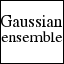Gaussian ensemble ``` OPGAUSSIAN(M,N,MODE) creates an M by N Gaussian ensemble operator. By setting MODE a number of different types of ensemble can be generated; MODE = 0 (default) explicitly creates an unnormalized matrix from the Normal distribution. MODE = 1 generates columns of the unnormalized matrix as the operator is applied. This allows for much larger ensembles since the matrix is implicit. For MODE = 2,3 columns are scaled to have unit Euclidean norm, when MODE = 3 the matrix is implicit. For MODE 4,5,6 the rows are orthogonalized using respectively the QR, ORTH and SVD functions. For the last three modes matrices are always generated explicitly. The orthogonalization modes work only when M < N. ```One-dimensional Haar wavelet ``` OPHAAR(N,LEVELS) creates a Haar wavelet operator for one dimensional signal of length N. LEVELS indicates the number of scales to use, default is 5. See also opWavelet ```Two-dimensional Haar wavelet ``` OPHAAR2D(N,LEVELS) creates a Haar wavelet operator for two dimensional signal of size M by N. LEVELS indicates the number of scales to use, default is 5. See also opWavelet ```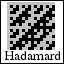Hadamard matrix ``` OPHADAMARD(N,NORMALIZED) creates a Hadamard operator for vectors of length N. When N is not a power of two, vectors are internally padded by zeroes to the next power of two. Multiplication is done using a fast recursive implementation. When the normalized flag is set, the columns of the Hadamard matrix are scaled to unit two-norm. By default the NORMALIZED flag is set to 0. ```Heaviside operator ``` OPHEAVISIDE(N,S) creates an operator for multiplication by an N by N Heaviside matrix. These matrices have ones below and on the diagonal and zeros elsewhere. S is a flag indicating whether the columns should be scaled to unit Euclidean norm. By default the columns are unnormalized. ```Kronecker tensor product ``` OPKRON(OP1,OP2) creates an operator that is the Kronecker tensor product of OP1 and OP2. ```Selection mask ``` OPMASK(A) creates an operator that computes the dot-product of a given vector with the (binary) mask provided by A. If A is a matrix it will be vectorized prior to use. See also opColRestrict, opRestriction. ```Apply arbitrary matrix as operator. ``` OPMATRIX(A,OPINFO) creates an operator that performs matrix-vector multiplication with matrix A. Optional parameter OPINFO can be used to override the default operator information returned when querying the operator, or provide a string giving the interpretation of the matrix. When OPINFO is a cell array the first entry is the operator name followed by additional information for that operator type (this option is mostly provided for internal use by other operators). ```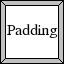Padding operator ``` OPPADDING(SIZELHS,SIZERHS) create an operator that pads and unpads matrices equally around each size. SIZELHS and SIZERHS give the matrix size before and after padding. SIZERHS must be greater than or equal to SIZELHS, and both must have an even number of rows and columns. ```Discard imaginary components ``` OPREAL(N) creates an operator that discards the imaginary components of input vectors of length N. ```Restriction operator ``` OPRESTRICTION(N,IDX) creates a restriction operator that selects the entries listed in IDX from an input vector of length N. The adjoint of the operator creates a vector of length N and fills the entries given by IDX with the input data. See also opColumnRestriction, opMask. ```Sign-ensemble operator. ``` OPSIGN(M,N,MODE) creates an M by N sign-ensemble operator. By setting MODE a number of different types of ensemble can be generated; MODE = 0 (default) explicitly creates an unnormalized matrix with random +1 and -1 entries. MODE = 1 generates columns of the unnormalized matrix as the operator is applied. This allows for much larger ensembles since the matrix is implicit. For MODE = 2,3 columns are scaled to have unit Euclidean norm, when MODE = 3 the matrix is implicit. ```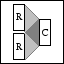Complex to real and imaginary ``` OPSPLITCOMPLEX(N) creates a nonlinear operator that splits a complex vector of length N into its real and imaginary parts [real(x); imag(x)]. In transpose mode it combines the real and imaginary parts into a complex vector. ```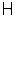Transpose operator ``` OPTRANSPOSE(OP) creates an operator that is the the adjoint operator OP. ```Wavelet operator ``` OPWAVELET(M,N,FAMILY,FILTER,LEVELS,TYPE) creates a wavelet operator of given FAMILY, for M by N matrices. The wavelet transformation is computed using the Rice Wavelet Toolbox. The remaining three parameters are optional. FILTER = 8 specifies the filter length and must be even. LEVELS = 5 gives the number of levels in the transformation. Both M and N must be divisible by 2^LEVELS. TYPE = 'min' indictates what type of solution is desired; 'min' for minimum phase, 'max' for maximum phase, and 'mid' for mid-phase solutions. ```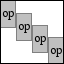Overcomplete windowed operator ``` OPWINDOWEDOP(M,OPERATOR,WINDOW,OVERLAP) creates an overcomplete windowed operator with overlap: / +------------+-------------------------+ | | | | | | Diag(W)*OP | | | | +------------+ | -- M / +------------+ | | -- OVERLAP \ | | Diag(W)*OP | | | | | +------------+ | | +------------+ | | | | Diag(W)*OP | \ +-------------------------+------------+ with W for WINDOW and OP for OPERATOR. Parameter OVERLAP must be strictly less than the length of WINDOW, which in turn must coincide with the number of rows in the OPERATOR. M indicates the signal length and prescribes the number of rows in the resulting operator. The number of columns is always an integer multiple of the number of columns in OPERATOR and depends on the required number of blocks. ```
(Last updated: 15-Feb-2008), Copyright 2007, University of British Columbia